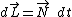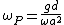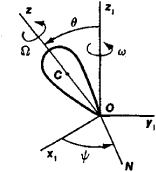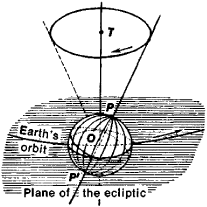# precession

(redirected from precessionally)
Also found in: Dictionary, Thesaurus, Medical.

## precession:

see gyroscopegyroscope
, symmetrical mass, usually a wheel, mounted so that it can spin about an axis in any direction. When spinning, the gyroscope has special properties. Many spinning objects exhibit some of these properties; the rotation of the earth about its axis gives it the
.

## Precession

The motion of an axis fixed in a body around a direction fixed in space. If the angle between the two is constant so that the axis sweeps out a circular cone, the motion is pure precession; oscillation of the angle is called nutation. An example of precession is the motion of the Earth's polar axis around the normal to the plane of the ecliptic; this is the precession of the equinoxes. A fast-spinning top, with nonvertical axis, which precesses slowly around the vertical direction, is another example. In both examples the precession is due to torque acting on the body. Another kind of precession, called free or fast precession, with a rate which is comparable to the rotation rate of the body, is seen, for instance, in a coin spun into the air.

As a simple example of gyroscopic motion, consider a rapidly spinning wheel with a horizontal axis supported at a distance d from the plane of the wheel (see illustration). The angular momentum is along the wheel symmetry axis and is approximately given by the angular momentum of the wheel about this axis; in the simple precession approximation to the motion, the angular momentum associated with precessional motion is neglected. The external torque due to the gravitational force is perpendicular to the wheel axis in the horizontal plane. The change in the angular momentum in an infinitesimal time interval dt is given by the rotational equation of motion in Eq. (1).

(1)See Rigid-body dynamics

Since dand are perpendicular, the length is unchanged to first order in dt. The direction of is rotated counterclockwise in the horizontal plane. The angular velocity of precession &ohgr;P about the vertical axis is then given by Eq. (2),

(2)where g is the gravitational acceleration, &ohgr; is the spin angular velocity, and a is the radius of the wheel. Thus &ohgr;P is independent of the mass of the wheel and inversely proportional to &ohgr;. For &ohgr; very large, the precession rate &ohgr;P is quite slow.

McGraw-Hill Concise Encyclopedia of Physics. © 2002 by The McGraw-Hill Companies, Inc.

## precession

(pri-sesh -ŏn) The slow periodic change in the direction of the axis of rotation of a spinning body due to the application of an external force (a torque). The extremities of the axis trace out circles in one complete precessional period if the rotational speed and applied torque are both constant. The Earth's axis precesses with a period of 25 800 years, the axial extremities, the celestial poles, tracing out circles about 23.5° in radius on the celestial sphere (see illustration). This radius is equal to the inclination of the Earth's equatorial plane to the plane of the ecliptic. The precession results primarily from the gravitational attraction of the Sun and Moon on the equatorial bulge of the nonspherical Earth. The Sun and Moon and also the planets pull on the bulge in an attempt to bring the equatorial plane into near coincidence with the plane of the ecliptic. The result of this gravitational action and the opposing rotation of the Earth is a precessional force that acts perpendicularly to both these actions.

The combined effect of the Sun and Moon leads to luni-solar precession, which together with the much smaller planetary precession gives the general precession of the Earth's axis and of the equinoxes. The annual general precession is given by

50.2564 + 0.0222T arcsecs
where T is the time in Julian centuries from 1900.0. Thus one precessional circle is completed about every 25 800 years, this period being the Platonic year. The precession of the Earth's axis leads not only to the precession of the equinoxes and the consequential change in stellar coordinates but also to the changing position of the celestial poles and hence to the slow change in the two pole stars.

Collins Dictionary of Astronomy © Market House Books Ltd, 2006
The following article is from The Great Soviet Encyclopedia (1979). It might be outdated or ideologically biased.

## Precession

the motion of a rigid body with a fixed point O that is composed of a rotation with an angular velocity Ω about the z-axis, which is rigidly attached to the body, and a rotation with an angular velocity ω about the z1-axis (see Figure 1). Here x1, y1, and z1 are the axes, conventionally called fixed, relative to which the body’s motion is considered, ON, called the line of nodes, is the line perpendicular to the plane z1O2, and ψ = ∠ x1ON is the angle of precession. In addition to precession the body also exhibits nutation, in which the angle of nutation θ = z1O2 changes (seeNUTATION).Figure 1. Diagram of precession

If θ is constant for the duration of the motion, that is, if nutation is absent, and the magnitudes of Ω and ω also remain constant, then the body’s motion is called steady precession. In this case, the z-axis describes a right circular cone about the z1-axis (the axis of precession). This type of precession is exhibited under any initial conditions by a symmetrical body fixed at its center of gravity (a gyroscope), on which no forces that would create a moment relative to the fixed point are acting. The axis of precession in this case is the constant direction of the body’s angular momentum (seeANGULAR MOMENTUM).

A symmetrical body fixed at an arbitrary point on its axis of symmetry and acted upon by gravity (a heavy gyroscope or top) exhibits precession about a vertical axis under any initial conditions. This precession is accompanied by nutational oscillations, whose amplitude and period decrease and whose frequency increases with an increase in the angular velocity of the rotation Ω. When Ω ≫ ω the apparent motion of the gyroscope differs little from steady precession; such precession is called pseudo-regular. The angular velocity of the pseudoregular precession of a heavy gyroscope is given approximately by the equation ω = Pa/I Ω, where P is the gyroscope’s weight, a is the distance between the fixed point and the center of gravity, and I is the gyroscope’s moment of inertia relative to its axis of symmetry.

S. M. TARG

In astronomy. In astronomy, precession refers to the slow motion of the earth’s axis of rotation along a circular cone, whose axis of symmetry is perpendicular to the plane of the ecliptic; this motion has a complete cycle of approximately 26,000 years. It is also called precession of the equinoxes, since it produces a slow shift in the vernal and autumnal equinoxes as a result of the motion of the planes of the ecliptic and the equator (Figure 2). The equinoxes are defined by the line of intersection of these two planes. More simply, precession can be viewed as a slow motion of the earth’s axis (the line parallel to the earth’s mean rotational axis PP°) about a circular cone whose axis is perpendicular to the ecliptic with a complete cycle of approximately 26,000 years.Figure 2. Precessional motion of the earth’s axis about a circular cone

The shift of the equinoxes proceeds along the ecliptic from east to west, that is, in a direction opposite to that of the apparent annual motion of the sun, at the rate of 50.3″ per year. As a result, the tropical year (the interval of time between two successive passages of the sun through the vernal equinox), which is linked to the succession of seasons on the earth, is shorter by 20 min 24 sec than the sidereal year (the period of one complete revolution of the earth around the sun). Because of precession, the ecliptic and equatorial coordinates of celestial bodies change (seeCELESTIAL COORDINATES). Stellar longitudes, which are measured from the vernal equinox, increase by 50.3″ per year, but latitudes do not change significantly. The right ascensions and declinations of stars change in a more complex manner.

As a result of precession, the pattern of the night sky’s diurnal rotation changes. Approximately 4,600 years ago the north celestial pole was near the star α Draconis. At present it is located near Polaris (α Ursae Minoris), and in 12,000 years the polestar will be Vega (α Lyrae). As the celestial pole moves among the stars, there is an associated change in the visibility of constellations in a given geographical area; this permits the approximate dating of ancient texts by means of references in them to one or another constellation.

The phenomenon of precession was discovered in the second century B.C. by the Greek astronomer Hipparchus, who compared stellar longitudes that he determined by observation with longitudes of the same stars recorded 150 years earlier by the Greek astronomers Timocharis and Aristyllos (Aristillus). An explanation of precession based on mechanics was first given by I. Newton in 1686. Newton regarded the earth, which is flattened at the poles, as a sphere engirdled by a ring about the equator; the sun more strongly attracts the half of the ring closer to it and thus tends to decrease the inclination of the equatorial plane of the earth to the plane of the ecliptic. A similar effect, but twice as strong and of a more complex nature, is caused by the moon. The combined effect of the attraction of the sun and the moon on the added equatorial mass of the rotating earth causes precession. Since the forces causing precession are continually changing as a result of the changing positions of the sun and the moon relative to the earth, small oscillations, which constitute nutation, are observed, in addition to the gradual shift of the vernal equinox—lunisolar precession.

Perturbations of the earth’s orbital motion resulting from the attraction of the other planets cause a small change in the orientation of the plane of the ecliptic in space; as a result, the inclination of the ecliptic to the equator decreases by 0.5” per year. The corresponding shift in the vernal equinox along the equator from west to east is called planetary precession. The overall motion of the vernal equinox, consisting of lunisolar precession and planetary precession, is called general precession. The theory of precession was basically developed in the 18th century by J. d’Alembert, P. Laplace, and L. Euler.

Accurate numerical values of the basic precessional quantities were first determined from observations by F. Bessel in the beginning of the 19th century; in 1841, O. V. Struve published new values. At the end of the 19th century S. Newcomb, in constructing a theory of the heliocentric and rotational motions of the earth, determined the values of the following precessional quantities: the lunisolar precession in declination (precession in declination does not depend on the attraction of the planets), the general precession in right ascension, the lunisolar precession in longitude, and the planetary precession in right ascension and in longitude.

The numerical values of the precessional quantities can be refined on the basis of statistical analysis of stellar proper motions, in which the apparent shift in the positions of stars caused by the motion of the sun in space and the rotation of our galaxy are taken into account. The most precise method for determining precessional quantities is based on the measurement of changes in the coordinates of galaxies, which in practice may be considered motionless objects as a result of their great distances from the earth. These measurements are part of an international program of work on the compilation of the Fundamental Catalog of Faint Stars; this work is being conducted on the initiative of Soviet astronomers (seeASTROMETRY).

### REFERENCES

Blazhko, S. N. Kurs sfericheskoi astronomii, 2nd ed. Moscow, 1954.
Kazakov, S. A. Kurs sfericheskoi astronomii, 2nd ed. Moscow-Leningrad, 1940.
“Reduktsionnye vychisleniia v astronomii.” In the book Astronomicheskii ezhegodnik SSSR na 1941 god. Moscow-Leningrad, 1940.

A. D. DUBIAGO AND V. K. ABALAKIN

## precession

[prē′sesh·ən]
(mechanics)
The angular velocity of the axis of spin of a spinning rigid body, which arises as a result of external torques acting on the body.
McGraw-Hill Dictionary of Scientific & Technical Terms, 6E, Copyright © 2003 by The McGraw-Hill Companies, Inc.

## gyro drift

The movement of a gyro axis in the horizontal plane caused by real or apparent drift. Also called gyro wander or precession. See gyro wander.